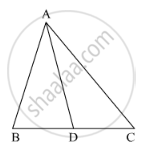Share

# In the Following Figure, Check Whether Ad is the Bisector of ∠A of ∆Abc in the Following Case: Ab = 6 Cm, Ac = 8 Cm, Bd = 1.5 Cm and Cd = 2 Cm - Mathematics

Course

#### Question

In the following figure, check whether AD is the bisector of ∠A of ∆ABC in the following case:

AB = 6 cm, AC = 8 cm, BD = 1.5 cm and CD = 2 cm#### Solution

It is given that, AB = 6 cm, AC = 8 cm, BD = 1.5 cm and CD = 2 cm.

We have to check whether AD is bisector of ∠A.

First we will check proportional ratio between sides.

So "AB"/"AC"="BD"/"DC"

rArr6/8=1.5/2

rArr3/4=3/4

(It is proportional)

Hence AD is bisector of ∠A.

Is there an error in this question or solution?

#### APPEARS IN

RD Sharma Solution for Class 10 Maths (2018 (Latest))
Chapter 7: Triangles
Ex. 7.3 | Q: 4.4 | Page no. 31
RD Sharma Solution for Class 10 Maths (2018 (Latest))
Chapter 7: Triangles
Ex. 7.3 | Q: 4.4 | Page no. 31